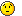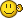## Recommended Posts

Hey guys,

I'm new to this forum and need your help...

I need to get the classnameNN or better the controlID from Checkboxes within a groupbox... I don't have any idea how to do this. Might i get some hints?Kind regards

Fabian

##### Share on other sites

Your best bet is to read the Help file on 'Controls' just search the Help file and it will outline how to do this. Hint: You'll need AutoIt Window Info Tool or Au3Info as it's known.

##### Share on other sites

Your best bet is to read the Help file on 'Controls' just search the Help file and it will outline how to do this. Hint: You'll need AutoIt Window Info Tool or Au3Info as it's known.

Already read this topic in the AutoIt help. And I also already tried to use the Window Info Programm but all it returns is 1 Button... It's not possible to select a single Checkbox in my groupboxNeed some more helpDon't know what I'm doing wrong, trying now for 1 hour haha

Here's what I get using Window Info on my groupbox. Sounds a bit wrong to me what he tells me##### Share on other sites

What's the program you're automating as perhaps the application has been discussed before.

It's called MEMSYS.

Here's a link to the website: http://www.videc.info/en/products/melsys-reporting-and-alarm-system

##### Share on other sites

Why not use ControlMove as perhaps the group control is overlapping the checkboxes and this is why you always get the group control instead of the checkboxes.

Edited by guinness

##### Share on other sites

I'm new to the AutoIt forum.

Came here to find solution to what I thought was an Autoit / 'Finder Tool' issue with controls in GroupBoxes that were used in my 3rd party COTS app.

I changed my theory because of the following experiment.

I created my own MS Windows program with controls in a GroupBox and Autoit / 'Finder Tool' found all the controls in the GroupBox

One of the developers in this or a similar discussion forum commented that the 'Finder Tool' Identified the GroupBox as a button class. That's exactly what I saw in my 3rd party app.

I had a thought... what if the 3rd party app developer had layered a button on a GroupBox and the controls that I could not access with the finder tool were actually on the button sitting on the groupbox boundary details. It might look like a groupbox container holding the subcontrols but actually they were top level windows form items sitting in the pixel footprint of the button.

I created another MS Windows program with some controls in a groupbox and some other controls on a large button.

Ran my program and used the AutoIt Finder Tool. It found all controls in the groupbox but could not find the buttons, textboxes, checkboxes, radiobuttons and listboxes that I placed on the button. Oddly, The 'Finder Tool' could see a combobox on the button.

My model for the problem has changed.

I think the Autoit finder tool knows the relative position(x,y) and geometry(w and h) of all controls on the form. They must be available in some form of list and the find tool moves the mouse controlled crosshair over the foreground window checking to see if it current position in the form corresponds to the rectangular footprint(x,y,w,h) of a control. When it does match, it fills in the control information fields for the match and does not continue searching for other controls that might also contain the mouse controlled crosshair position. This would make any controls below the button's x,y,w,h position invisible to the Autoit finder tool.

Is there any way to just see the control ID information for all controls contained within the form and their x,y.w,h geometries?

Thanks!

##### Share on other sites

My signature has a function to grab all controls, and output them to your console (as long as they are standard windows controls)...it's gives class name, id, position, visibility, text, and some other stuff...pass in the handle for the window.

I've had the same issue with the spy tool, where it would only grab the control group, and not allow me to select the specific controls within it...that's why I wrote up this function: to get some identifier to use in automation scripts, reliably.

Simple example:

```\$h = WinGetHandle("[CLASS:Notepad]")
If Not IsHWnd(\$h) Then
EndIf

GetAllWindowsControls(\$h)```

Output:

```Func=[GetAllWindowsControls]: ControlCounter=[  1] ControlID=[   15] Handle=[0x002D0068] ClassNN=[              Edit1] XPos=[   0] YPos=[   0] Width= Height=[ 582] IsVisible= Text=[].
Func=[GetAllWindowsControls]: ControlCounter=[  2] ControlID=[ 1025] Handle=[0x000D0528] ClassNN=[msctls_statusbar321] XPos=[   0] YPos=[ 559] Width= Height=[  23] IsVisible= Text=[].```

Edit...this one flexes the muscles a bit more:

```\$h = WinGetHandle("[CLASS:CabinetWClass]")
If Not IsHWnd(\$h) Then
Run("explorer")
\$h = WinWait("[CLASS:CabinetWClass]","",5000)
EndIf

GetAllWindowsControls(\$h)```

Output:

```Func=[GetAllWindowsControls]: ControlCounter=[  1] ControlID= Handle=[0x0021061A] ClassNN=[ Address Band Root1] XPos=[ 105] YPos=[  57] Width=[ 793] Height=[  28] IsVisible= Text=[].
Func=[GetAllWindowsControls]: ControlCounter=[  2] ControlID=[    0] Handle=[0x0020070C] ClassNN=[ Breadcrumb Parent1] XPos=[ 107] YPos=[  61] Width=[ 755] Height=[  22] IsVisible= Text=[].
Func=[GetAllWindowsControls]: ControlCounter=[  3] ControlID=[    0] Handle=[0x00110796] ClassNN=[            Button1] XPos=[ 172] YPos=[ 102] Width=[   0] Height=[   0] IsVisible= Text=[].
Func=[GetAllWindowsControls]: ControlCounter=[  4] ControlID=[    0] Handle=[0x002707CC] ClassNN=[            Button2] XPos=[ 172] YPos=[ 102] Width=[   0] Height=[   0] IsVisible= Text=[].
Func=[GetAllWindowsControls]: ControlCounter=[  5] ControlID=[    0] Handle=[0x000C07DA] ClassNN=[    CtrlNotifySink1] XPos=[   0] YPos=[  90] Width=[ 156] Height=[ 631] IsVisible= Text=[].
Func=[GetAllWindowsControls]: ControlCounter=[  6] ControlID=[    0] Handle=[0x002307F2] ClassNN=[    CtrlNotifySink2] XPos=[ 172] YPos=[ 102] Width=[   0] Height=[   0] IsVisible= Text=[].
Func=[GetAllWindowsControls]: ControlCounter=[  7] ControlID=[    0] Handle=[0x000D064E] ClassNN=[    CtrlNotifySink3] XPos=[ 172] YPos=[ 102] Width=[   0] Height=[   0] IsVisible= Text=[].
Func=[GetAllWindowsControls]: ControlCounter=[  8] ControlID=[    0] Handle=[0x000C080A] ClassNN=[    CtrlNotifySink4] XPos=[   0] YPos=[  90] Width=[   0] Height=[   0] IsVisible= Text=[].
Func=[GetAllWindowsControls]: ControlCounter=[  9] ControlID=[    0] Handle=[0x000C064A] ClassNN=[    CtrlNotifySink5] XPos=[ 160] YPos=[  90] Width=[ 963] Height=[ 631] IsVisible= Text=[].
Func=[GetAllWindowsControls]: ControlCounter=[ 10] ControlID=[    0] Handle=[0x001C01DE] ClassNN=[    CtrlNotifySink6] XPos=[ 160] YPos=[  90] Width=[   0] Height=[   0] IsVisible= Text=[].
Func=[GetAllWindowsControls]: ControlCounter=[ 11] ControlID=[    0] Handle=[0x000C0610] ClassNN=[    CtrlNotifySink7] XPos= YPos=[  90] Width=[  17] Height=[ 631] IsVisible= Text=[].
Func=[GetAllWindowsControls]: ControlCounter=[ 12] ControlID=[    0] Handle=[0x001C0798] ClassNN=[      DirectUIHWND1] XPos=[ 913] YPos=[  60] Width=[ 194] Height=[  24] IsVisible= Text=[].
Func=[GetAllWindowsControls]: ControlCounter=[ 13] ControlID=[    0] Handle=[0x000E0664] ClassNN=[      DirectUIHWND2] XPos=[   0] YPos=[  90] Width= Height=[ 654] IsVisible= Text=[].
Func=[GetAllWindowsControls]: ControlCounter=[ 14] ControlID=[    0] Handle=[0x0019067E] ClassNN=[      DirectUIHWND3] XPos=[ 160] YPos=[  90] Width=[ 963] Height=[ 631] IsVisible= Text=[].
Func=[GetAllWindowsControls]: ControlCounter=[ 15] ControlID=[    0] Handle=[0x0017028E] ClassNN=[DUIViewWndClassName1] XPos=[   0] YPos=[  90] Width= Height=[ 654] IsVisible= Text=[].
Func=[GetAllWindowsControls]: ControlCounter=[ 16] ControlID=[    0] Handle=[0x00210660] ClassNN=[ msctls_progress321] XPos=[ 107] YPos=[  61] Width=[ 789] Height=[  22] IsVisible= Text=[].
Func=[GetAllWindowsControls]: ControlCounter=[ 17] ControlID= Handle=[0x001205E4] ClassNN=[msctls_statusbar321] XPos=[   0] YPos=[  67] Width=[   0] Height=[  23] IsVisible= Text=[].
Func=[GetAllWindowsControls]: ControlCounter=[ 18] ControlID=[    0] Handle=[0x000A0790] ClassNN=[NamespaceTreeControl1] XPos=[   0] YPos=[  90] Width=[ 156] Height=[ 631] IsVisible= Text=[Namespace Tree Control].
Func=[GetAllWindowsControls]: ControlCounter=[ 19] ControlID=[    0] Handle=[0x000B0512] ClassNN=[         NetUIHWND1] XPos=[   0] YPos=[   0] Width= Height=[  55] IsVisible= Text=[].
Func=[GetAllWindowsControls]: ControlCounter=[ 20] ControlID=[    0] Handle=[0x0022058E] ClassNN=[           NUIPane1] XPos=[   0] YPos=[  31] Width= Height=[  55] IsVisible= Text=[].
Func=[GetAllWindowsControls]: ControlCounter=[ 21] ControlID= Handle=[0x001402E8] ClassNN=[     ReBarWindow321] XPos=[   0] YPos=[  55] Width= Height=[  35] IsVisible= Text=[].
Func=[GetAllWindowsControls]: ControlCounter=[ 22] ControlID= Handle=[0x00190554] ClassNN=[     ReBarWindow322] XPos=[   0] YPos=[  90] Width= Height=[   0] IsVisible= Text=[].
Func=[GetAllWindowsControls]: ControlCounter=[ 23] ControlID=[    0] Handle=[0x000D0670] ClassNN=[         ScrollBar1] XPos=[ 160] YPos=[  90] Width=[   0] Height=[   0] IsVisible= Text=[].
Func=[GetAllWindowsControls]: ControlCounter=[ 24] ControlID=[    0] Handle=[0x000F06A2] ClassNN=[         ScrollBar2] XPos= YPos=[  90] Width=[  17] Height=[ 631] IsVisible= Text=[].
Func=[GetAllWindowsControls]: ControlCounter=[ 25] ControlID=[    0] Handle=[0x001905BE] ClassNN=[        Search Box1] XPos=[ 908] YPos=[  60] Width=[ 204] Height=[  24] IsVisible= Text=[].
Func=[GetAllWindowsControls]: ControlCounter=[ 26] ControlID=[    0] Handle=[0x002B028A] ClassNN=[SearchEditBoxWrapperClass1] XPos=[ 913] YPos=[  60] Width=[ 194] Height=[  24] IsVisible= Text=[].
Func=[GetAllWindowsControls]: ControlCounter=[ 27] ControlID=[    0] Handle=[0x001407B0] ClassNN=[     SeparatorBand1] XPos=[ 902] YPos=[  70] Width=[   6] Height=[   1] IsVisible= Text=[].
Func=[GetAllWindowsControls]: ControlCounter=[ 28] ControlID=[    0] Handle=[0x00180580] ClassNN=[     SeparatorBand2] XPos= YPos=[  70] Width=[   7] Height=[   1] IsVisible= Text=[].
Func=[GetAllWindowsControls]: ControlCounter=[ 29] ControlID=[    0] Handle=[0x00190522] ClassNN=[Shell Preview Extension Host1] XPos=[   0] YPos=[  90] Width=[   0] Height=[   0] IsVisible= Text=[Shell Preview Extension Host].
Func=[GetAllWindowsControls]: ControlCounter=[ 30] ControlID=[    0] Handle=[0x0039076C] ClassNN=[  SHELLDLL_DefView1] XPos=[ 160] YPos=[  90] Width=[ 963] Height=[ 631] IsVisible= Text=[ShellView].
Func=[GetAllWindowsControls]: ControlCounter=[ 31] ControlID=[    0] Handle=[0x001105BA] ClassNN=[ShellTabWindowClass1] XPos=[   0] YPos=[  90] Width= Height=[ 654] IsVisible= Text=[File Explorer].
Func=[GetAllWindowsControls]: ControlCounter=[ 32] ControlID=[    0] Handle=[0x000D0690] ClassNN=[            Static1] XPos=[   0] YPos=[  90] Width=[   0] Height=[   0] IsVisible= Text=[Namespace Tree Control].
Func=[GetAllWindowsControls]: ControlCounter=[ 33] ControlID=[  100] Handle=[0x000E0638] ClassNN=[     SysTreeView321] XPos=[   0] YPos=[  90] Width=[ 156] Height=[ 631] IsVisible= Text=[Tree View].
Func=[GetAllWindowsControls]: ControlCounter=[ 34] ControlID=[    0] Handle=[0x001D062E] ClassNN=[   ToolbarWindow321] XPos=[   4] YPos=[  57] Width=[  77] Height=[  30] IsVisible= Text=[Navigation buttons].
Func=[GetAllWindowsControls]: ControlCounter=[ 35] ControlID=[    0] Handle=[0x001E08CC] ClassNN=[   ToolbarWindow322] XPos=[  81] YPos=[  60] Width=[  24] Height=[  29] IsVisible= Text=[Up band toolbar].
Func=[GetAllWindowsControls]: ControlCounter=[ 36] ControlID=[ 1001] Handle=[0x001402EC] ClassNN=[   ToolbarWindow323] XPos=[ 107] YPos=[  61] Width=[ 755] Height=[  22] IsVisible= Text=[Address: Quick access].
Func=[GetAllWindowsControls]: ControlCounter=[ 37] ControlID=[    0] Handle=[0x002A05A8] ClassNN=[   ToolbarWindow324] XPos=[ 862] YPos=[  61] Width=[  34] Height=[  22] IsVisible= Text=[Address band toolbar].
Func=[GetAllWindowsControls]: ControlCounter=[ 38] ControlID=[    0] Handle=[0x001605D4] ClassNN=[   ToolbarWindow325] XPos=[   2] YPos=[  90] Width= Height=[   0] IsVisible= Text=[].
Func=[GetAllWindowsControls]: ControlCounter=[ 39] ControlID=[    0] Handle=[0x001B080E] ClassNN=[        TravelBand1] XPos=[   0] YPos=[  55] Width=[  81] Height=[  32] IsVisible= Text=[].
Func=[GetAllWindowsControls]: ControlCounter=[ 40] ControlID=[    0] Handle=[0x000D077E] ClassNN=[UIRibbonCommandBar1] XPos=[   0] YPos=[  31] Width= Height=[  24] IsVisible= Text=[Ribbon].
Func=[GetAllWindowsControls]: ControlCounter=[ 41] ControlID=[    0] Handle=[0x00140646] ClassNN=[UIRibbonCommandBarDock1] XPos=[   0] YPos=[  55] Width=[   0] Height=[ 689] IsVisible= Text=[UIRibbonDockLeft].
Func=[GetAllWindowsControls]: ControlCounter=[ 42] ControlID=[    0] Handle=[0x00140674] ClassNN=[UIRibbonCommandBarDock2] XPos= YPos=[  55] Width=[   0] Height=[ 689] IsVisible= Text=[UIRibbonDockRight].
Func=[GetAllWindowsControls]: ControlCounter=[ 43] ControlID=[    0] Handle=[0x000E064C] ClassNN=[UIRibbonCommandBarDock3] XPos=[   0] YPos=[  31] Width= Height=[  24] IsVisible= Text=[UIRibbonDockTop].
Func=[GetAllWindowsControls]: ControlCounter=[ 44] ControlID=[    0] Handle=[0x000E0526] ClassNN=[UIRibbonCommandBarDock4] XPos=[   0] YPos=[ 744] Width= Height=[   0] IsVisible= Text=[UIRibbonDockBottom].
Func=[GetAllWindowsControls]: ControlCounter=[ 45] ControlID=[    0] Handle=[0x000B062C] ClassNN=[  UIRibbonWorkPane1] XPos=[   0] YPos=[  31] Width= Height=[  24] IsVisible= Text=[Ribbon].
Func=[GetAllWindowsControls]: ControlCounter=[ 46] ControlID=[    0] Handle=[0x001605CA] ClassNN=[  UIRibbonWorkPane2] XPos=[   0] YPos=[   0] Width=[ 100] Height=[ 100] IsVisible= Text=[UIRibbonWorkPane].
Func=[GetAllWindowsControls]: ControlCounter=[ 47] ControlID=[    0] Handle=[0x002304EA] ClassNN=[UniversalSearchBand1] XPos=[ 908] YPos=[  57] Width=[ 204] Height=[  28] IsVisible= Text=[].
Func=[GetAllWindowsControls]: ControlCounter=[ 48] ControlID=[    0] Handle=[0x004804E6] ClassNN=[            UpBand1] XPos=[  81] YPos=[  56] Width=[  24] Height=[  29] IsVisible= Text=[].
Func=[GetAllWindowsControls]: ControlCounter=[ 49] ControlID=[    0] Handle=[0x002005A6] ClassNN=[           WorkerW1] XPos=[   0] YPos=[  55] Width= Height=[  35] IsVisible= Text=[].
Func=[GetAllWindowsControls]: ControlCounter=[ 50] ControlID= Handle=[0x001206FE] ClassNN=[           WorkerW2] XPos=[   0] YPos=[  90] Width= Height=[   0] IsVisible= Text=[].```

Edited by jdelaney

IEbyXPATH-Grab IE DOM objects by XPATH IEscriptRecord-Makings of an IE script recorder ExcelFromXML-Create Excel docs without excel installed GetAllWindowControls-Output all control data on a given window.

## Create an account

Register a new account

• ### Recently Browsing   0 members

×

• Wiki

• Back

• #### Beta

• Git
• FAQ
• Our Picks
×
• Create New...